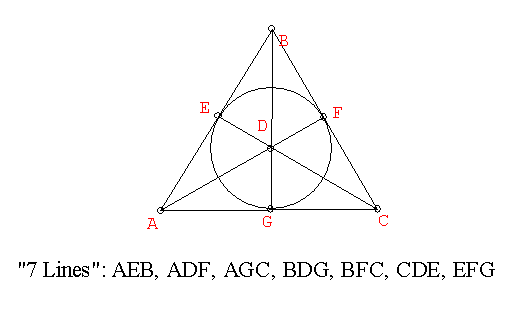Connecting Axioms to Models.
We can visualize the seven point- seven line geometry using the following figure:• It is apparent from this figure that 7 points are enough to satisfy the axioms for the seven point geometric structure described in the reference, and that any more points would force a violation of one of the axioms.
You should work through this argument yourself.  Suppose there is an eighth point, H.
Then there must be an eighth line determined by A and H. [why?]
But can AH have a point in common with the line with points BCF?
No... can you see why having a point in common with BCF would be contrary to the axioms. Which axioms would be violated?

In a geometry structure we use a language with words like "point" and "line" for objects and relations between these objects like "is on"  or "passes through" and operations on these objects like "meet", "intersect" and "join".

In the structure initially we list certain properties (axioms) of the objects that are supposed as "true", and we use logic to infer (deduce) other properties (theorems) of the objects.

A (set theoretic) interpretation for a geometry structure is a set in which the words of the geometry stucture's language are identified with set elements and sets and relations and operations on sets.

If any (and every) true statement (theorem) about the mathematical structure is also true of the corresponding statement  about the interpretation, then the interpretation is called a model for the geometric structure.

Thus we can say that an interpretation for the geometric structure described previously with point and lines is given by a set of objects labelled A,B,C,D,E,F, and G.
Points correspond to the objects.
Lines correspond to sets of three objects from one of the seven previously described sets:  {A,E,B}, {A,D,F}... {E,F,G} .
A point is on a line if the object is an element of the line set.
This interpretation is a model and it is visualized by the previous "7 line" triangular figure.

Another interpretation for a geometric structure might involve just 3 objects, A,B and C, for points and three lines {A,B}, {A,C}, and {B,C}.
This might be represented by  the three vertices of a triangle.

This geometry does not satisfy the first axiom for the previously described geometric structure, because a line does not have three distinct points.
It does satisfy the other three axioms.  So this interpretation is not a model for that structure!

However, there is something to be learned about that structure from the two interpretations we have discussed.

From the first model with the 7 points and 7 lines we can see that the axioms for the structure are consistent in the sense that it is not possible to derive a logical contradiction from these axioms. [If such a contradiction could be logically inferred, then the model would have to exhibit this contradiction!]

From the second model with the 3 points and 3 lines we can see that a collection of axioms based on the negation of the first axiom but otherwise the same as the axioms for the geometric structure are also consistent.

Since both the first axiom and it negation appear to be consistent with the other axioms, it is not possible to infer the first axiom or its negation from the other axioms.

Thus the existence of the two different models allows us to infer that the first axiom  (and it's negation) cannot be logically inferred from the other axioms, i.e., the first axiom is independent  of the other axioms.